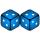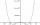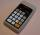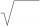# Power + square root - examples

1. PoolIf water flows into the pool by two inlets, fill the whole for 8 hours. The first inlet filled pool 6 hour longer than second. How long pool take to fill with two inlets separately?
2. TV transmitterThe volume of water in the rectangular swimming pool is 6998.4 hectoliters. The promotional leaflet states that if we wanted all the pool water to flow into a regular quadrangle with a base edge equal to the average depth of the pool, the prism would have.
3. Circle chordWhat is the length d of the chord circle of diameter 36 m, if the distance from the center circle is 16 m?
4. Rectangular cuboidThe rectangular cuboid has a surface area 5334 cm2, its dimensions are in the ratio 2:4:5. Find the volume of this rectangular cuboid.
5. Cube diagonalDetermine length of the cube diagonal with edge 75 mm.
6. R TrapeziumRectangular trapezium has bases 12 and 5 and area 84 cm2. What is its perimeter?
7. Prism XThe prism with the edges of the lengths x cm, 2x cm and 3x cm has volume 20250 cm3. What is the area of surface of the prism?
8. RhombusFind the length of the other diagonal and area of rhombus. The perimeter of a rhombus is 40 cm and one of the diagonals is of length 10 cm.
9. BallsThree metal balls with volumes V1=71 cm3 V2=78 cm3 and V3=64 cm3 melted into one ball. Determine it's surface area.
10. Event probabilityThe probability of event P in 8 independent experiments is 0.33. What is the probability that the event P occurs in one experiment (probability is same)?
11. Geometric sequence 3In geometric sequence is a8 = 312500; a11= 39062500; sn=1953124. Calculate the first item a1, quotient q and n - number of members by their sum s_n.By introducing a new variable solve biquadratic equation: ?
13. Chord ABWhat is the length of the chord AB if its distance from the center S of the circle k(S, 92 cm) is 10 cm?
14. ExpIf ?, then n is:
15. Cube 6Volume of the cube is 216 cm3, calculate its surface area.
16. Digits A, B, CFor the various digits A, B, C is true: the square root of the BC is equal to the A and sum B+C is equal to A. Calculate A + 2B + 3C. (BC is a two-digit number, not a product).
17. 3d vector componentThe vector u = (3.9, u3) and the length of the vector u is 12. What is is u3?
18. RootsCalculate the square root of these numbers:
19. Geometric sequenceIn the geometric sequence is a4 = 20 a9 = -160. Calculate the first member a1 and quotient q.
20. PowerNumber ?. Find the value of x.

Do you have an interesting mathematical example that you can't solve it? Enter it, and we can try to solve it.

To this e-mail address, we will reply solution; solved examples are also published here. Please enter e-mail correctly and check whether you don't have a full mailbox.